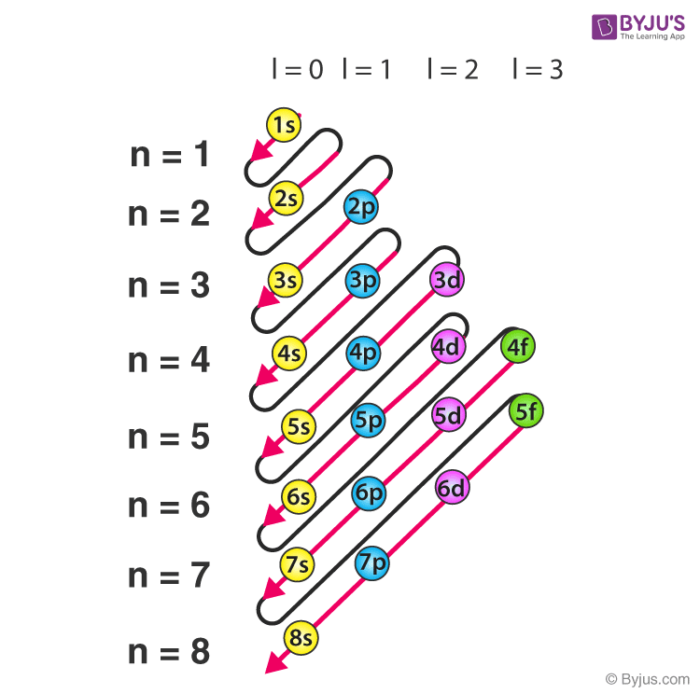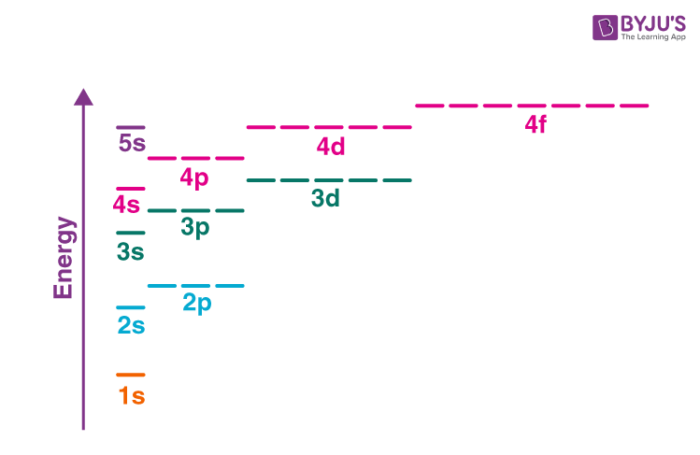# Aufbau Principle

## What is the Aufbau Principle?

The Aufbau principle dictates the manner in which electrons are filled in the atomic orbitals of an atom in its ground state.

It states that electrons are filled into atomic orbitals in the increasing order of orbital energy level. According to the Aufbau principle, the available atomic orbitals with the lowest energy levels are occupied before those with higher energy levels.

The word ‘Aufbau’ has German roots and can be roughly translated as ‘construct’ or ‘build up’. A diagram illustrating the order in which atomic orbitals are filled is provided below. Here, ‘n’ refers to the principal quantum number and ‘l’ is the azimuthal quantum number.The Aufbau principle can be used to understand the location of electrons in an atom and their corresponding energy levels. For example, carbon has 6 electrons and its electronic configuration is 1s22s22p2.

It is important to note that each orbital can hold a maximum of two electrons (as per the Pauli exclusion principle). Also, the manner in which electrons are filled into orbitals in a single subshell must follow Hund’s rule, i.e. every orbital in a given subshell must be singly occupied by electrons before any two electrons pair up in an orbital.

## Salient Features of the Aufbau Principle

• According to the Aufbau principle, electrons first occupy those orbitals whose energy is the lowest. This implies that the electrons enter the orbitals having higher energies only when orbitals with lower energies have been completely filled.
• The order in which the energy of orbitals increases can be determined with the help of the (n+l) rule, where the sum of the principal and azimuthal quantum numbers determines the energy level of the orbital.
• Lower (n+l) values correspond to lower orbital energies. If two orbitals share equal (n+l) values, the orbital with the lower n value is said to have lower energy associated with it.
• The order in which the orbitals are filled with electrons is: 1s, 2s, 2p, 3s, 3p, 4s, 3d, 4p, 5s, 4d, 5p, 6s, 4f, 5d, 6p, 7s, 5f, 6d, 7p, and so on.

## Exceptions

The electron configuration of chromium is [Ar]3d54s1 and not [Ar]3d44s2 (as suggested by the Aufbau principle). This exception is attributed to several factors such as the increased stability provided by half-filled subshells and the relatively low energy gap between the 3d and the 4s subshells.

The energy gap between the different subshells is illustrated below.Half filled subshells feature lower electron-electron repulsions in the orbitals, thereby increasing the stability. Similarly, completely filled subshells also increase the stability of the atom. Therefore, the electron configurations of some atoms disobey the Aufbau principle (depending on the energy gap between the orbitals).

For example, copper is another exception to this principle with an electronic configuration corresponding to [Ar]3d104s1. This can be explained by the stability provided by a completely filled 3d subshell.

## Electronic Configuration using the Aufbau Principle

### Writing the Electron Configuration of Sulphur

• The atomic number of sulphur is 16, implying that it holds a total of 16 electrons.
• As per the Aufbau principle, two of these electrons are present in the 1s subshell, eight of them are present in the 2s and 2p subshell, and the remaining are distributed into the 3s and 3p subshells.
• Therefore, the electron configuration of sulphur can be written as 1s22s22p63s23p4.

### Writing the Electron Configuration of Nitrogen

• The element nitrogen has 7 electrons (since its atomic number is 7).
• The electrons are filled into the 1s, 2s, and 2p orbitals.
• The electron configuration of nitrogen can be written as 1s22s22p3

## Electronic ConfigurationTest your knowledge on aufbau principle!